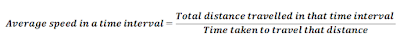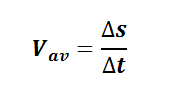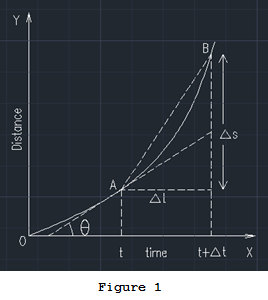### Average Speed and Instantaneous Speed

The average speed of a particle in a time interval is defined as the distance travelled by a particle divided by the time interval to cover that distance.For example, if you are travelling from your home to school by bus, suppose the bus has 30km and taken 1 hr to reach the school. Then you can tell to your friend that, my bus has an average speed of 30km/hr from my home to school. However, if you are noticing speedometer readings, the bus has different speed in different time (is called instantaneous speed, will discuss soon).
Hence, we conclude that, the average speed gives the overall rapidity with which particle moves in this interval. Through this you can represent overall travel to a significant value which describes how speed he has come to destination.Instantaneous speed of a particle is the speed at a particular time. This is actually the reading shown in the speedometer. Let ∆s be the distance travelled in the time interval t to t+∆t. The average speed in this time interval is, Vav

Suppose ∆t is vanishingly small, the distance travelled in this interval ∆s will also be very small. But the value of  ∆s/∆t will be a finite value which is the instantaneous speed ( or simply, speed) at that time.
Graphical interpretation:
Distance versus time graph :
Let figure 1 shown below be the distance verses time graph of a particle. Also A be the distance of particle at time t from the origin O and B be the distance of particle at time t+∆t from the origin O.Then the slop of the curve at point corresponding to a time will give instantaneous speed. This means, the speed at A is the slope of the curve at A.
If we want to find the average speed between to point, say A and B, we should find the slop of the line connecting these two points (chord AB in the figure above).
As ∆t approaches zero, the chord AB becomes the tangent at A, and the average speed becomes instantaneous speed at A.
Speed versus time graph:
If you plot speed at each time in Y axis and time in X axis, you will get a curve as shown in figure 2 below. Actually we can generate this curve from figure 2, by taking the instantaneous speed (or slop at each point) at all time. So you got the idea, how we will get the distance from the speed versus time graph, right? Yes, Integrating the curve in the required time interval will get distance. This means, area under the curve from t1 to t2 as shown in figure 2 will get the distance travelled by the particle in that time interval.

Dimension of speed is LT-1
Unit of speed is meter/sec.